The flow of electric charges through a conductor constitutes an electric current. Quantitatively, the electric current in a conductor across an area held perpendicular to the direction of flow of charge is defined as the amount of charge flowing across that area per unit time.

If a charge ∆Q passes through an area in time t to t + ∆t, then the current I at time t is given by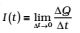If the current is steady i.e., the rate of flow of charge does not change with time, then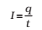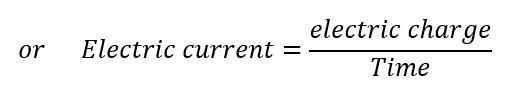where q is the charge that flows across the given area in time t.

#### SI unit of current is ampere (A).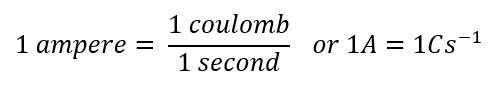### Electric Currents in Conductor

An electric charge will experience a force if an electric field is applied. If it is free to move, it will thus move contributing to a current. In nature, free charged particles do exist like in upper strata of the atmosphere called the ionosphere. In some materials, the electrons will still be bound, i.e., they will not accelerate even if an electric field is applied. In other materials, notably metals, some of the electrons are practically free to move within the bulk material. These materials, generally called conductors, develop electric currents in them when an electric field is applied.

The charges particles whose flow in a definite direction constitutes the electric current are called current carriers.

In solid conductor: free valence electrons are the charge carriers

In liquid: Negatively and positively charged ions are the charge carriers

In gas: Positive ions and the electrons are the charge carriers.

If we consider solid conductors, then, of course, the atoms are tightly bound to each other so that the current is carried by the negatively charged electrons. There are, however, other types of conductors like electrolytic solutions where positive and negative charges both can move.

Consider first the case when no electric field is present. The electrons will be moving due to thermal motion during which they collide with the fixed ions. An electron colliding with an ion emerges with the same speed as before the collision. However, the direction of its velocity after the collision is completely random. This is called thermal velocity of an electron.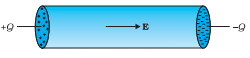At a given time, there is no preferential direction for the velocities of the electrons. Thus on the average, the number of electrons travelling in any direction will be equal to the number of electrons travelling in the opposite direction. So, there will be no net electric current.

If an electric field is applied, the electrons will be accelerated due to this field towards +Q. The electrons as long as they are moving will constitute an electric current. The current for a very short duration is called as transient Current.